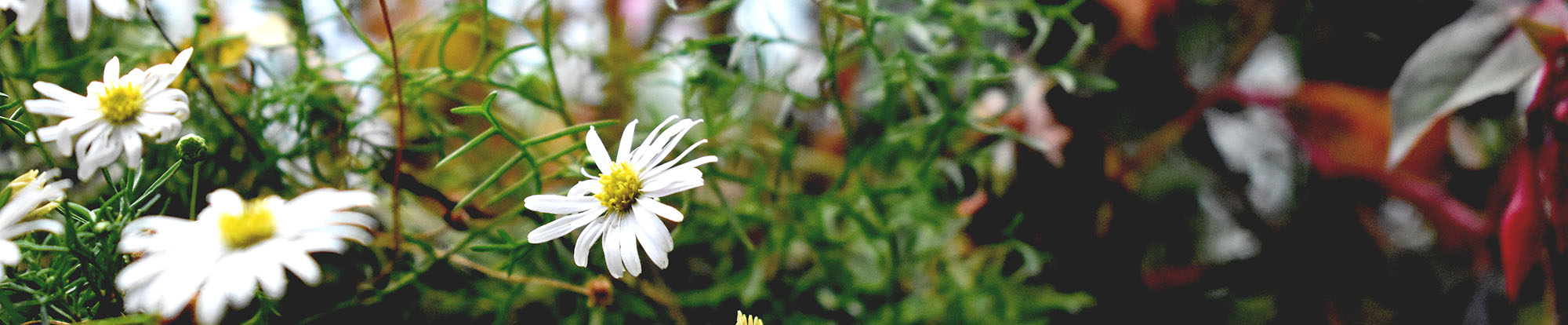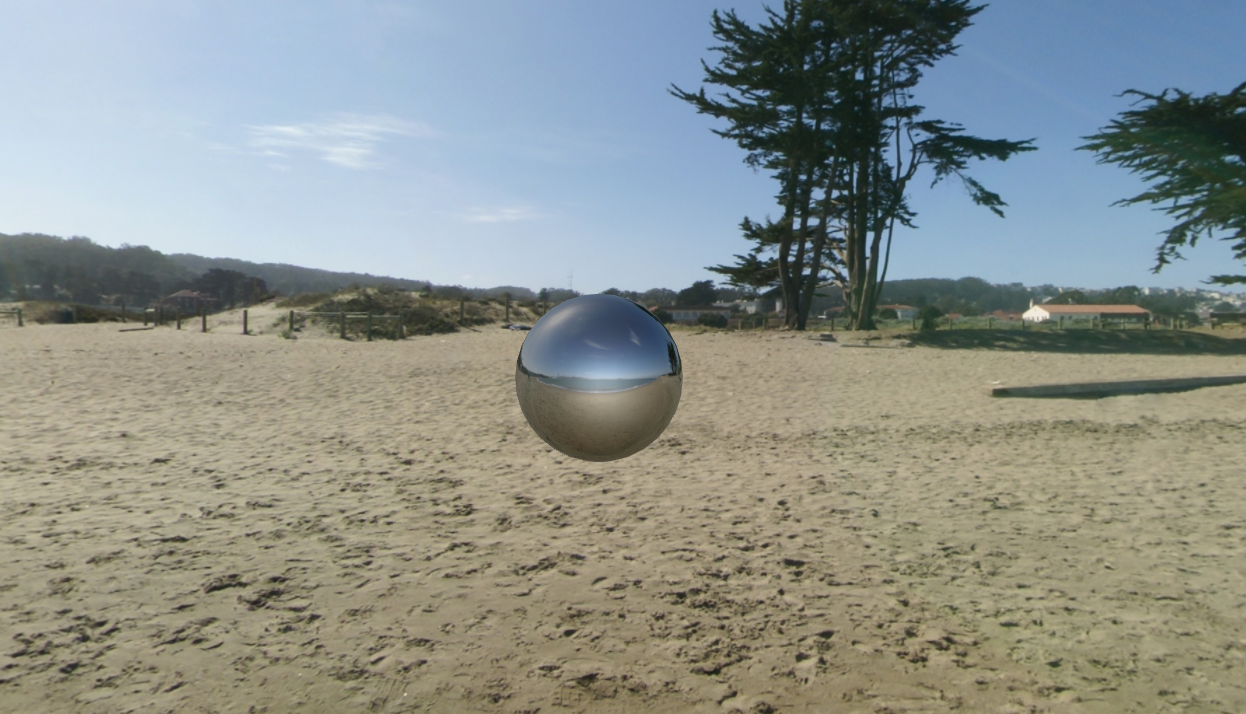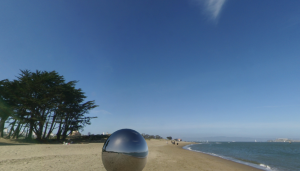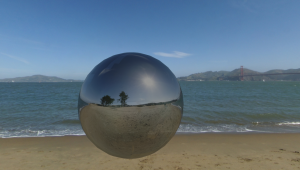# OpenGL 4 Environment Mapping

I’ve been building on my OpenGL 4 and GLSL knowledge by implementing a 3D application featuring a cubemapped skybox and a sphere showing a reflection of the skybox. The shader for the sphere also uses the Blinn-Phong model for illumination, however I’ve set the ratio of the environment colour to be higher than the lighting, so it’s not overly visible.

Skybox Set up

I’ve used SOIL for image loading, and fortunately it provides a method for loading cubemaps. Here is my method for initialising the skybox:

``````void SkyBox::Init(){
glActiveTexture(GL_TEXTURE0);
glEnable(GL_TEXTURE_CUBE_MAP);
glGenTextures(1, &cubemap);
glBindTexture(GL_TEXTURE_CUBE_MAP, cubemap);
glEnable(GL_BLEND);

glTexParameteri(GL_TEXTURE_CUBE_MAP, GL_GENERATE_MIPMAP, GL_TRUE);
glTexParameteri(GL_TEXTURE_CUBE_MAP, GL_TEXTURE_MAG_FILTER, GL_NEAREST);
glTexParameteri(GL_TEXTURE_CUBE_MAP, GL_TEXTURE_MIN_FILTER, GL_NEAREST);
glTexParameteri(GL_TEXTURE_CUBE_MAP, GL_TEXTURE_WRAP_S, GL_CLAMP_TO_EDGE);
glTexParameteri(GL_TEXTURE_CUBE_MAP, GL_TEXTURE_WRAP_T, GL_CLAMP_TO_EDGE);
glTexParameteri(GL_TEXTURE_CUBE_MAP, GL_TEXTURE_WRAP_R, GL_CLAMP_TO_EDGE);

glTexGeni(GL_S, GL_TEXTURE_GEN_MODE, GL_REFLECTION_MAP); //texture coordinate generation
glTexGeni(GL_T, GL_TEXTURE_GEN_MODE, GL_REFLECTION_MAP);
glTexGeni(GL_R, GL_TEXTURE_GEN_MODE, GL_REFLECTION_MAP);

glEnable(GL_TEXTURE_GEN_S);
glEnable(GL_TEXTURE_GEN_T);
glEnable(GL_TEXTURE_GEN_R);

"models/skybox/posx.jpg",
"models/skybox/posy.jpg",
"models/skybox/negy.jpg",
"models/skybox/negz.jpg",
"models/skybox/posz.jpg",
SOIL_CREATE_NEW_ID,
SOIL_FLAG_MIPMAPS);

}``````I found these parameters gave me a nice seamless box with no visible aliasing. I also created a method to initially send some data to the shader: the parts that aren’t going to change. These include the positions of the vertices, the cubemap texture and the triangle indices (which are used to indicate which vertices make up each triangle).

``````void SkyBox::SendToShader(GLuint shaderID){
/******************************************************************/
// THIS METHOD SENDS THE GEOMETRY OF THE OBJECT TO THE SHADER. //
// THIS INCLUDES THE VERTICES, THE TEXTURE COORDS AND THE COLOURS //
// AS THEY DON'T CHANGE. IT GETS CALLED ONCE PER OBJECT. //
/*****************************************************************/

// VAO allocation
glGenVertexArrays(1, &vaoID);
glBindVertexArray(vaoID);

//initialise VBO for vertices
glGenBuffers(1, &vboID);
glBindBuffer(GL_ARRAY_BUFFER, vboID);
glBufferData(GL_ARRAY_BUFFER, numOfVerts*3*sizeof(GLfloat), verts, GL_STATIC_DRAW);

//set VBO pointer and vertex attributes for VAO
//first param is 0 because thats the layout ID, you'd use e.g. "in_Verts" if not using layouts
glEnableVertexAttribArray(0);
glVertexAttribPointer(0, 3, GL_FLOAT, GL_FALSE, 0, 0);

//initialise VBO for triangle indices
glGenBuffers(1, &triangleIndicesBO);
glBindBuffer(GL_ELEMENT_ARRAY_BUFFER, triangleIndicesBO);
glBufferData(GL_ELEMENT_ARRAY_BUFFER, numOfTris * 3 * sizeof(unsigned int), tris, GL_STATIC_DRAW);

//allocate memory for cubemap to send to shader
glActiveTexture(GL_TEXTURE0);
glEnable(GL_TEXTURE_CUBE_MAP);
glEnable(GL_TEXTURE_CUBE_MAP_SEAMLESS);
glBindTexture(GL_TEXTURE_CUBE_MAP, cubemap);

//unbind
glBindVertexArray(0);
glBindBuffer(GL_ARRAY_BUFFER, 0);
glBindBuffer(GL_ELEMENT_ARRAY_BUFFER, 0);

}``````

Skybox Update Method

My third method int eh Skybox class is called every frame, and sends the matrices to the shader. The view matrix consists of the rotation part of the main camera matrix used in the application, this means that when the first-person camera is rotated, the view of the skybox also changes. We do not need the translation part, because the skybox is drawn “infinitely far away”, so that it makes the environment feel bigger (this is also why GL_DEPTH_TEST is disabled).

``````void SkyBox::Display(GLuint shaderID, glm::mat4 Projection, glm::mat4 Camera){

/**************************************************************************/
// THIS METHOD SENDS THE VARIOUS MATRICES TO THE SHADER. //
// IT GETS CALLED ONCE PER OBJECT, PER FRAME. //
/*************************************************************************/
glClear(GL_COLOR_BUFFER_BIT | GL_DEPTH_BUFFER_BIT);

glDisable(GL_DEPTH_TEST);
glCullFace(GL_FRONT);

View = glm::mat4(glm::mat3(Camera)); //get rotation part only of camera matrix

//draw objects
glBindVertexArray(vaoID); // select VAO
glDrawElements(GL_TRIANGLES, numOfTris*3, GL_UNSIGNED_INT, 0);
glBindVertexArray(0); //unbind the vertex array object

glUseProgram(0);
glEnable(GL_DEPTH_TEST);
glCullFace(GL_BACK);
glFlush();
}``````

The actual shaders are very simple, because we do not need lighting, only the correct parts of the cubemap to be sampled.

``````#version 400
layout (location = 0) in vec3 vertexPos;
uniform mat4 ModelView;
uniform mat4 Projection;
uniform mat4 View;

out vec3 tex_Coords;

void main(void)
{
gl_Position = Projection * View * ModelView * vec4(vertexPos, 1.0);
//gl_Position = Projection * ModelView * vec4(vertexPos, 1.0);
//gl_Position = vec4(vertexPos, 1.0);
tex_Coords = vertexPos;
}
[/sourcecode]

[sourcecode language="cpp" wraplines="false" collapse="false"]
#version 400
in vec3 tex_Coords;
out vec4 out_Colour; //colour for the pixel
uniform samplerCube cubemap;

void main(void)
{
out_Colour = texture(cubemap, tex_Coords);
//out_Colour = vec4(1.0,0.0,0.0,1.0); //red
//out_Colour = vec4(tex_Coords,1.0); //show texture coords
}``````

The parts I have commented out I tend to use for testing purposes when setting the skybox up; my first port of call will always be to just get something displaying on the screen, even if it’s only a colour!

The shaders I wrote for the sphere are a little more complicated. In the vertex shader, the vertices and normals are transformed from model space to world space.

``````#version 400

layout (location = 0) in vec3 vertexPos;
layout (location = 1) in vec3 vertexNorm;

uniform mat4 ModelView;
uniform mat4 Projection;
uniform mat4 View;
uniform mat3 NormalMatrix;

out vec3 ex_Normal;
out vec3 ex_VertPos;
out vec3 ex_LightDir;
out vec3 halfVec;

void main(void)
{
gl_Position = Projection * View * ModelView * vec4(vertexPos, 1.0);
ex_Normal = normalize(mat3(transpose(inverse(ModelView))) * vertexNorm); //for reflection
ex_VertPos = vec3(ModelView * vec4(vertexPos, 1.0));

//divide the light dir by a radius, large number = large surface area affected
ex_LightDir = (vec3(0.0, 12.0, 60.0) - ex_VertPos) /radius1; //remove -ex_VertPos for dir light
vec3 surfaceToEye = normalize(-ex_VertPos);
halfVec = normalize(ex_LightDir + surfaceToEye);
}``````

The fragment shader involves the calculation of the ambient, diffuse and specular components of the light, along with the reflection mapping. The equations for the first three can be found on
this page, and the calculations involved in working out the reflection can be found here. The cube is textured using a sample of the cubemap, calculated by the reflected direction from the camera view point and the vertex normal.

``````#version 400

in vec3 ex_Normal;
in vec3 ex_VertPos;
in vec3 ex_LightDir;
in vec2 ex_UV;
in vec3 halfVec;

uniform vec3 eyePos;
uniform samplerCube cubeMap;

out vec4 out_Colour; //colour for the pixel

void main(void)
{
vec4 light_ambient = vec4(0.2, 0.2, 0.2, 1.0);
vec4 light_diffuse = vec4(0.7, 0.7, 0.7, 1.0);
vec4 light_specular = vec4(0.6, 0.6, 0.6, 1.0);
vec4 material_ambient = vec4(0.1, 0.1, 0.1, 1.0);
vec4 material_diffuse = vec4(0.7, 0.7, 0.7, 1.0);
vec4 material_specular = vec4(1.0, 1.0, 1.0, 1.0);

vec4 ambientProduct = light_ambient * material_ambient;
vec4 diffuseProduct = light_diffuse * material_diffuse;
vec4 specularProduct = light_specular * material_specular;

float LdotN, HdotN, spec, att;
vec4 Illumination;
vec3 L;

float shininess = 10.0;

att = max(0.0, 1.0 - dot(ex_LightDir, ex_LightDir));
L = normalize(ex_LightDir);

LdotN = max(dot(L, ex_Normal2), 0.0);
HdotN = max(dot(halfVec, ex_Normal2), 0.0);
spec = pow(HdotN, shininess);

Illumination = att * ambientProduct;
Illumination += att * diffuseProduct * LdotN;
Illumination += att * specularProduct * spec;

vec4 lightColour = Illumination;

vec3 incident = normalize(ex_VertPos - eyePos);
vec3 reflectDir = reflect(incident, normalize(ex_Normal2));

vec4 envColour = texture(cubeMap, reflectDir); //reflection part
envColour = mix(envColour, lightColour, 0.4); //x*(1-a) + y*a
out_Colour = envColour;
}
``````

I have chosen to show more of the environment than the lighting colour, to make the sphere look like it has a shinier material.In my application, the user can move around the sphere and “look around” in any direction using an xbox 360 controller.

Scroll to top